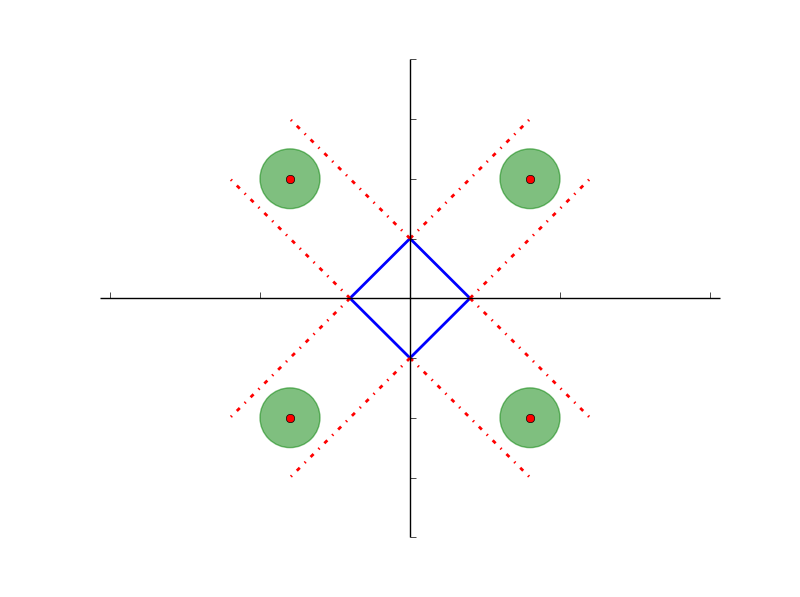人脸识别（稀疏表示、人工神经网络）

1. 稀疏表示

minαAg(α)=Δf(α)+λΩ(α)

Ω(α)=α0

f(α)$f(\alpha)$ 为忠诚项，通常取平方误差：

f(α)=12xDα22

minαA=12xDα22+λα1

minαA=12xDα22+λα2

l2$l_2$ 正则项具有收缩作用，但不具有选择功能，即所有变量都会被选择，而与 Tikhonov 正则相比，Lasso 具有收缩和选择的双重功能，Lasso 中的 l1$l_1$ 范数能够产生稀疏的解，可参考：范数2. 组合 Lasso

x=Dα=[D1D2...Dp]α1α2...αp

minαA=12xDiαi22+λi=1pαi1

3. 稀疏表示人脸识别

1. 由所有样本构成字典 D=[D1,D2,...,DK]$D=[D_1,D_2,...,D_K]$，其中Di$D_i$为第 i 类样本组成的子矩阵。
2. 归一化矩阵D，使每列的 l2$l_2$ 范数为 1
3. 求解稀疏表示问题，计算测试样本 x 在第 i 类上的重构误差：
ri(x)=xDiαi2
4. 依据重构误差确定样本类别：
c(x)=argminiri(x)

4. 基于人工神经网络的人脸识别

en=12(yW21H)2

1. 网络初始化：

1. 根据输入输出序列（X，Y）确定：
• 网络输入层节点数 n（不考虑额外的偏置项为样本长度，如果考虑单元个数需要+1）
• 隐含层结点数 l
• 输出层节点数 m
2. 初始化：
• 初始化输入层与隐藏层的之间的权值 wj,i,0<jl;0<in$w_{j,i},0，可以组成矩阵 W10$W_{10}$
• 初始化隐藏层与输出层之间的权值 wk,j,0<kn;0<jl$w_{k,j},0，可以组成矩阵 W21$W_{21}$
• 给定学习速率 η$\eta$
• 给定神经元激励函数：σ(y)=11+ey$\sigma \left( y \right) = \frac{1}{{1 + {e^{ - y}}}}$ （本文采用）或 σ(y)=eyeyey+ey=tanh(y)$\sigma \left( y \right) = \frac{{{e^y} - {e^{ - y}}}}{{{e^y} + {e^{ - y}}}} = \tanh \left( y \right)$
2. 隐藏层输出计算：
随机选择一个样本 （x，y），计算隐含层输出 H：

Hl×1=σ(W10xn×1)

3. 输出层输出计算：
根据隐藏层的输出 H，计算 BP 神经网络的预测输出 O：
Om×1=W21Hl×1
4. 误差计算：
根据网络的预测输出 O 和期望输出 y，计算网络的预测误差 eO：
eO=ym×1Om×1
5. 对于网络的输出单元，计算它们的误差项：
δ(L)jδ(L)=enH(L)j=(yiOi)=(ym×1Om×1)=eO
6. 对于网络的隐藏层单元，计算它们的误差项：
δ(l)jδ(l)=k=1d(l+1)((δ(l+1)k)(w(l+1)kj)(σ(H(l)j)))=σ(H(l)).(WT21δ(L))l×1
7. 更新每个网络权重：
wjiW21W10=wji+Δwji=wji+ηδjxji=W21+ηδ(L)HT=W10+ηδ(l)xT

5. 结果

K 近邻分类：        精度 = 70.72%；重构时间为： 0.76s

7. 参考文献

1. 《视觉机器学习20讲》
2. 人工神经网络-文本识别
3. 《Robust face recognition via sparse representation》